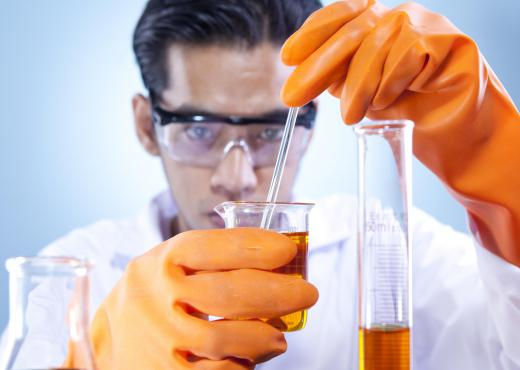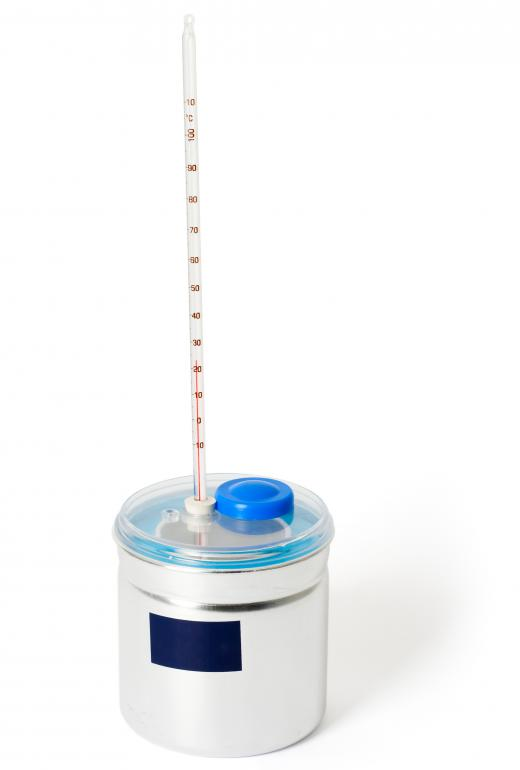Science
Fact Checked

# What is Enthalpy?

Victoria Blackburn
Victoria Blackburn

Enthalpy is the heat energy exchange that takes place during chemical reactions. It has the symbol H and is measured in kJ/mol, or kilojoules per mole. The energy exchanged with the surrounding environment at constant pressure is called the enthalpy change of a reaction. To measure the change, standard conditions are used, including a pressure of 1 atmosphere and a temperature of 298 Kelvin (77°F or 25°C).

When the reaction is giving off heat, it is said to be exothermic. In this situation, the enthalpy change is negative, since the reaction is going from high energy to low energy due to the loss of heat energy to its surroundings. If energy flows from the surrounding environment into a system, or heat is being taken in, it is said to be endothermic. In this instance, the change is positive as the system is gaining energy in the form of heat.Enthalpy refers to the heat energy exchange that occurs during chemical reactions.

Energy level diagrams are the most common way of showing such changes in heat. There are two general diagrams used, one for exothermic reactions and one for endothermic reactions. For an exothermic reaction, the energy of the reactants of the reaction would be higher than the energy of the products resulting. The opposite is true for endothermic reactions. The change is clearly marked on both diagrams.A simple calorimeter can be used to measure a change in enthalpy.

Two ways of measuring a change in enthalpy are a simple calorimeter or a simple flame calorimeter. A simple calorimeter is made of a container of water, with heat transferred into the water. The change in temperature of the water that is recorded will be due to the energy exchange of the reaction. When a simple calorimeter is used, the reaction takes place within the water in the calorimeter itself.

With a simple flame calorimeter, the reaction takes place outside of the device. During the reaction, the heat has to be transferred to the water inside of the calorimeter. This type of calorimeter is used for measuring the change of combustion reactions.

In order to calculate the change in enthalpy, three things must be known. The first is a constant, which is the specific heat capacity of the water, which is 4.2 J/g/°C. The mass of the water and the amount of the temperature change of the water must be measured. The formula for calculating this change is 4.2 x mass of water x temperature change.

## You might also Likeanon937659

Can someone explain what is enthalpy relaxation?

anon332215

I wanted to know how allowing the water to boil when measuring temperature change affects the accuracy of the enthalpy change, in the experiment of heating water with a spirit burner.

anon323632

What are some uses of enthalpy?

anon139717

cougars: I wanted to ask is the freezing of water to ice an exothermic reaction? it means the enthalpy change is negative? is it true that enthalpy change is the amount of energy (total internal and external) of the system? So what exactly is enthalpy change?

submariner

@ Chicada- I would also like to add a little bit to cougars' explanation of how to calculate enthalpy change in phase changes. One thing that often confuses people is the fact the freezing of water to ice is actually an exothermic reaction. Most people assume that an exothermic reaction is one that is hot, and they ask how this can be when ice is cold. The truth is the water is giving off heat when it is cooled to ice. Water is one of those rare substances that form a solid below room temperature, so we associate this exothermic process with cold rather than a system giving off heat.

Having said that, the inverse of what Cougars explained is true. Phase changes in the reverse direction are exothermic, and have a negative change in enthalpy. When thinking about enthalpy, it is always best to think of the reaction in terms of the system and not the surroundings. This is the best way to avoid confusion and understand how the whole process works.

cougars

@ Chicada- This is one of the easier concepts related to enthalpy to understand, but it can still be very confusing at first. When looking at a phase change from liquid to vapor, the enthalpy change is positive. Here is a real example. In the phase change from water to steam, the enthalpy change is positive because energy must be input into the water to create the phase change. One must boil the water to create steam. The phase change, on the other hand, is endothermic because the water is the system, and one must input heat into the system.

The phase changes of a solid to a liquid and a solid to a gas are positive as well. In all of these cases, energy must be input into the system to propagate the phase change, thus resulting in an endothermic reaction.